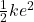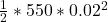## A force of 44 N will stretch a rubber band 88 cm ​(0.080.08 ​m). Assuming that​ Hooke’s law​ applies, how far will aa 11​-N force stretch th

Question

A force of 44 N will stretch a rubber band 88 cm ​(0.080.08 ​m). Assuming that​ Hooke’s law​ applies, how far will aa 11​-N force stretch the rubber​ band? How much work does it take to stretch the rubber band this​ far?

in progress 0
5 months 2021-08-18T07:51:20+00:00 1 Answers 9 views 0

## Answers ( )

The rubber band will be stretched 0.02 m.

The work done in stretching is 0.11 J.

Explanation:

Force 1 = 44 N

extension of rubber band = 0.080 m

Force 2 = 11 N

extension = ?

According to Hooke’s Law, force applied is proportional to the extension provided elastic limit is not extended.

F = ke

where k = constant of elasticity

e = extension of the material

F = force applied.

For the first case,

44 = 0.080K

K = 44/0.080 = 550 N/m

For the second situation involving the same rubber band

Force = 11 N

e = 550 N/m

11 = 550e

extension e = 11/550 = 0.02 m

The work done to stretch the rubber band this far is equal to the potential energy stored within the rubber due to the stretch. This is in line with energy conservation.

potential energy stored ===>= 0.11 J